# 基于曲梁弹性理论的弯曲覆岩变形及应力分析*

(1. 菏泽学院 城市建设学院， 山东 菏泽 274015；2. 中国矿业大学 力学与土木工程学院， 江苏 徐州 221116)

## 1 用位移函数表示的曲梁弹性解答

### 1.1 位移函数的控制方程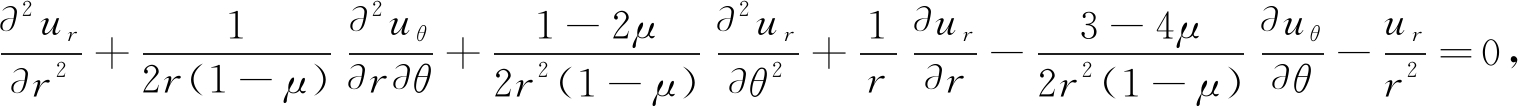(1a)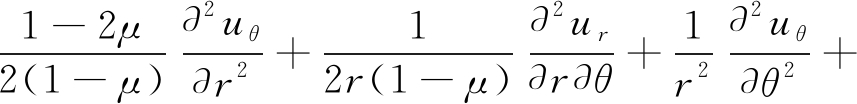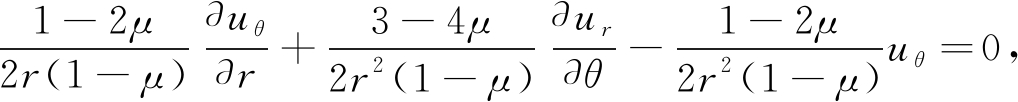(1b)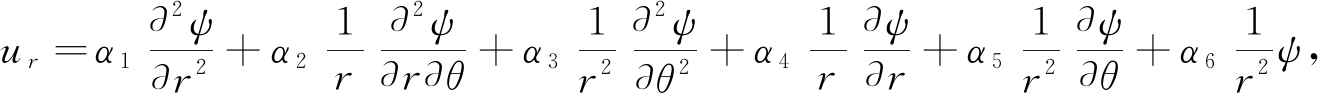(2a)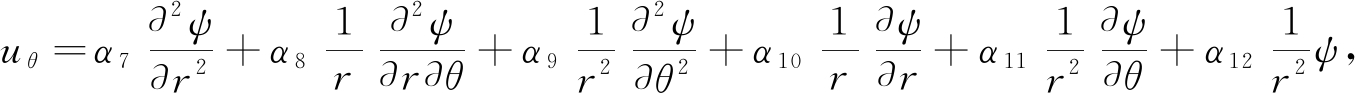(2b)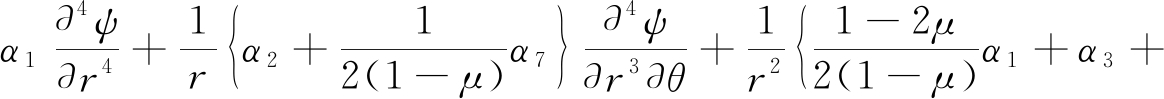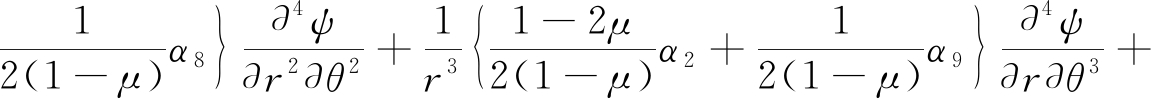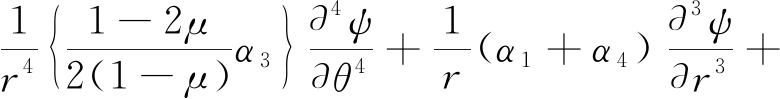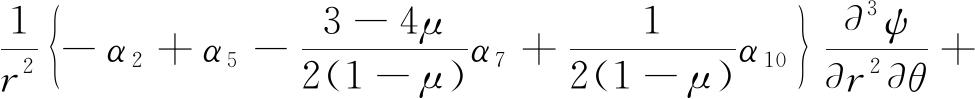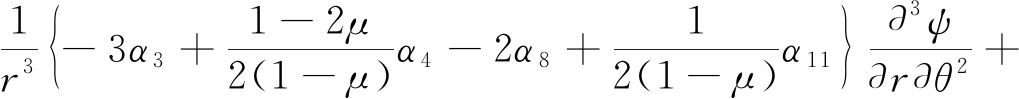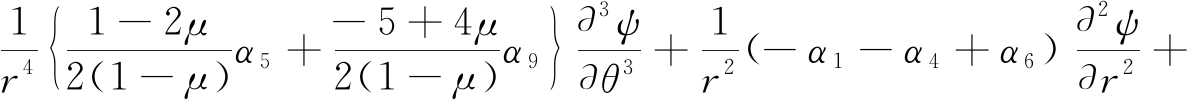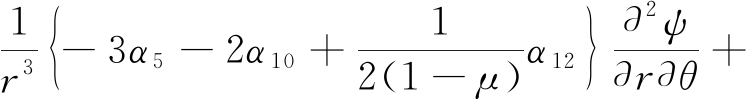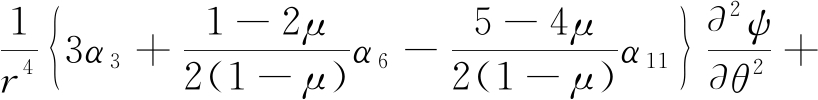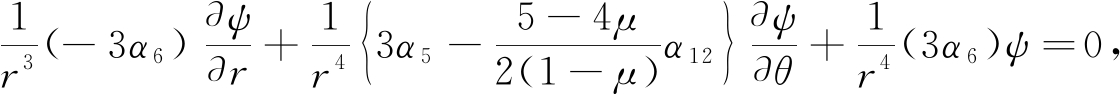(3a)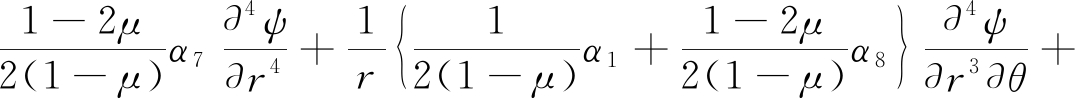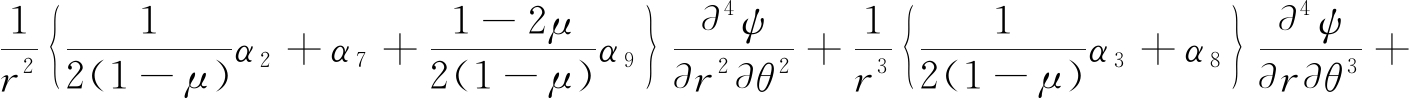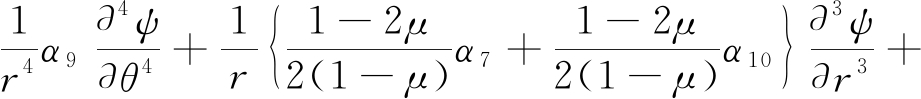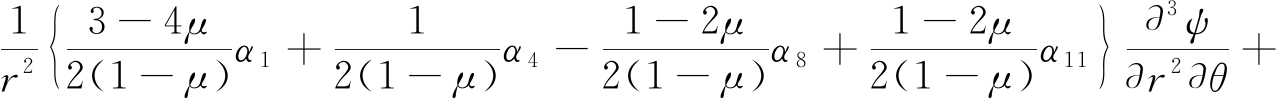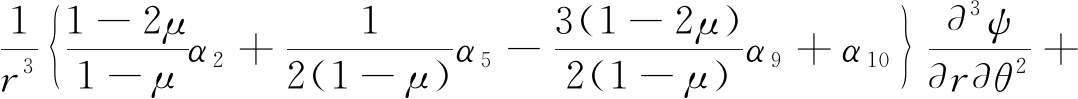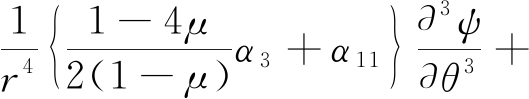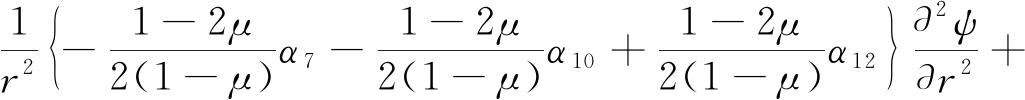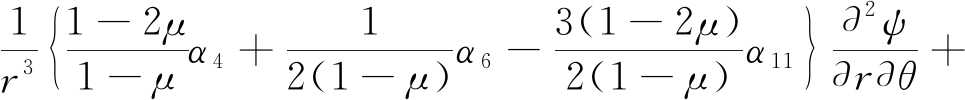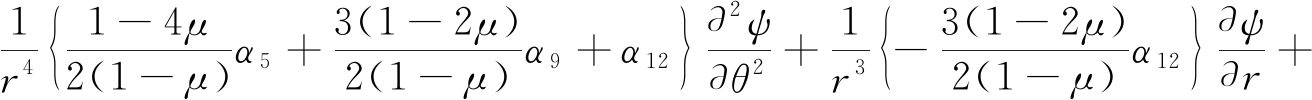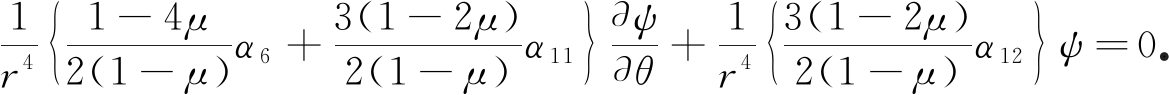(3b)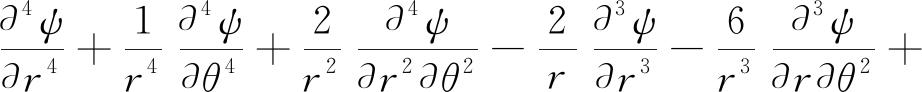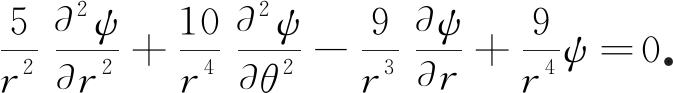(4)

### 1.2 用位移函数表示的位移分量和应力分量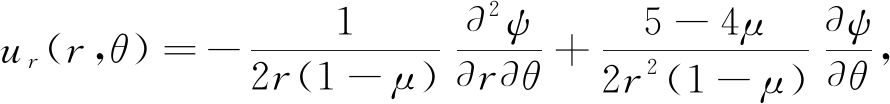(5a)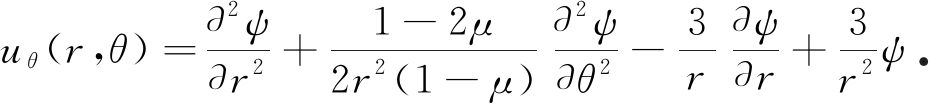(5b)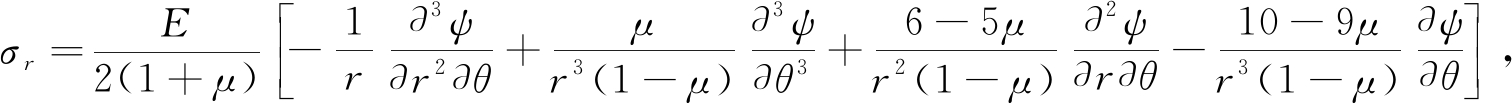(6a)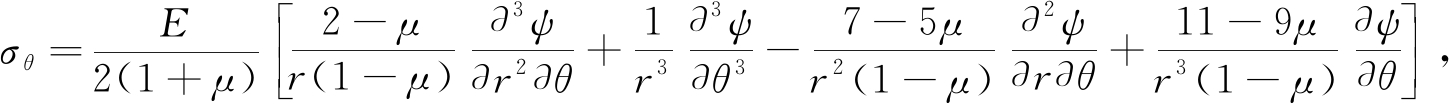(6b)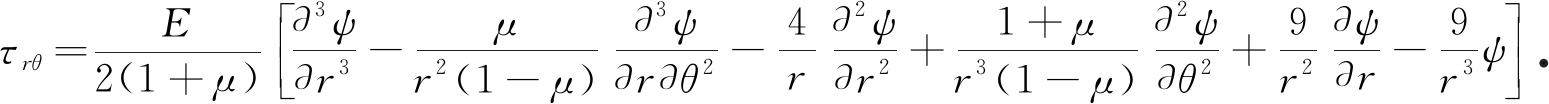(6c)

### 1.3 差分原理

1.3.1 控制方程的差分格式

ξ1ψ(i+2, j)+ξ2ψ(i+1, j+1)+ξ3ψ(i+1, j)+ξ2ψ(i+1, j-1)+

ξ4ψ(i, j+2)+ξ5ψ(i, j+1)+ξ6ψ(i, j)+ξ5ψ(i, j-1)+

ξ4ψ(i, j-2)+ξ7ψ(i-1, j+1)+ξ8ψ(i-1, j)+

ξ7ψ(i-1, j-1)+ξ9ψ(i-2, j)=0，

(7)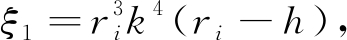ξ2=rih2k2(2ri-3h),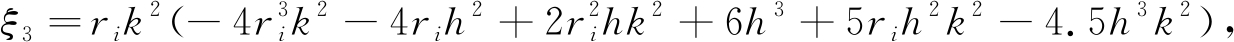ξ4=h4,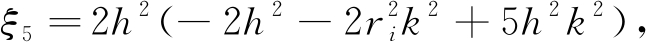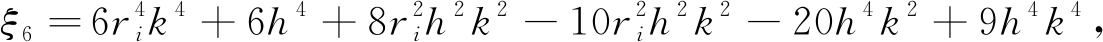ξ7=rih2k2(2ri+3h),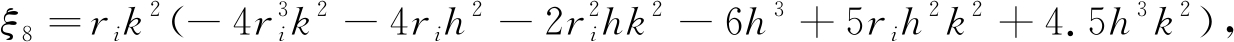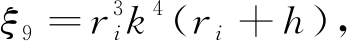hk分别为r方向、θ方向的网格长度．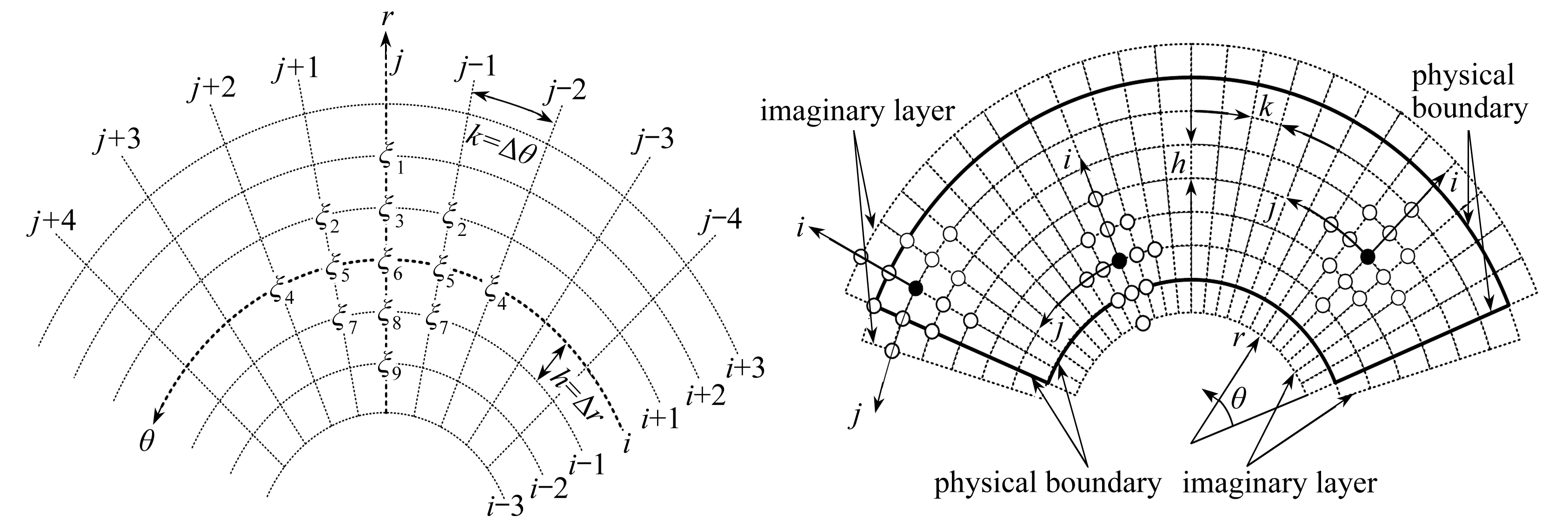Fig. 1 The finite difference scheme of the governing equation

1.3.2 位移分量的差分格式

(a) r-向前差分，θ-向前差分

ur(i, j)=a1ψ(i+2, j+2)-4a1ψ(i+2, j+1)+3a1ψ(i+2, j)-

4a1ψ(i+1, j+2)+16a1ψ(i+1, j+1)-12a1ψ(i+1, j)+

(3a1-b1)ψ(i, j+2)-(12a1-4b1)ψ(i, j+1)+

(9a1-3b1)ψ(i, j)；

(8a)

(b) r-向前差分，θ-向后差分

ur(i, j)=-3a1ψ(i+2, j)+4a1ψ(i+2, j-1)-a1ψ(i+2, j-2)+

12a1ψ(i+1, j)-16a1ψ(i+1, j-1)+4a1ψ(i+1, j-2)-

(9a1-3b1)ψ(i, j)+(12a1-4b1)ψ(i, j-1)-

(3a1-b1)ψ(i, j-2)；

(8b)

(c) r-向后差分，θ-向前差分

ur(i, j)=-(3a1+b1)ψ(i, j+2)+(12a1+4b1)ψ(i, j+1)-

(9a1+3b1)ψ(i, j)+4a1ψ(i-1, j+2)-16a1ψ(i-1, j+1)+

12a1ψ(i-1, j)-a1ψ(i-2, j+2)+

4a1ψ(i-2, j+1)-3a1ψ(i-2, j)；

(8c)

(d) r-向后差分，θ-向后差分

ur(i, j)=(9a1+3b1)ψ(i, j)-(12a1+4b1)ψ(i, j-1)+

(3a1+b1)ψ(i, j-2)-12a1ψ(i-1, j)+16a1ψ(i-1, j-1)-

4a1ψ(i-1, j-2)+3a1ψ(i-2, j)-

4a1ψ(i-2, j-1)+a1ψ(i-2, j-2)，

(8d)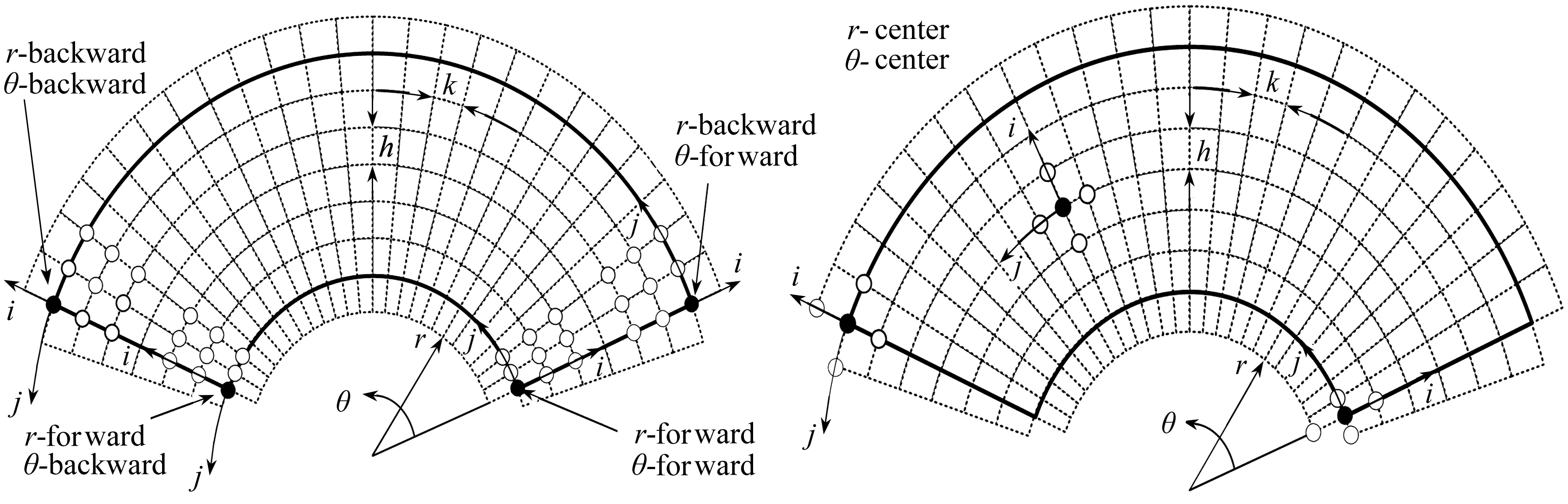Fig. 2 The finite difference scheme of the Fig. 3 The finite difference scheme of the radial displacement circumferential displacement

uθ(i, j)=(a2+c2)ψ(i+1, j)+b2ψ(i, j+1)+

(-2a2-2b2+d2)ψ(i, j)+b2ψ(i, j-1)+(a2-c2)ψ(i-1, j)，

(9)

1.3.3 应力分量的差分格式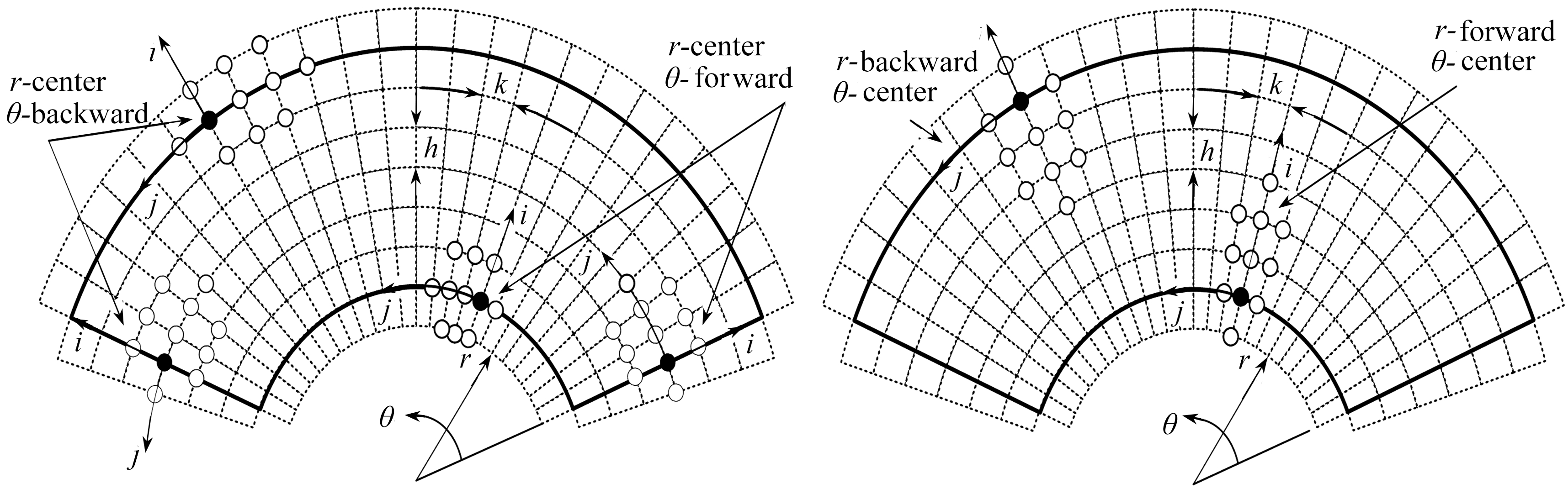Fig. 4 The finite difference scheme of the radial Fig. 5 The finite difference scheme and circumferential stresses of the shear stress

1) 径向应力分量σr的差分方程

(a) r-中心差分，θ-向前差分

σr(i, j)=(-A1-C1)ψ(i+1, j+2)+(4A1+4C1)ψ(i+1, j+1)+

(-3A1-3C1)ψ(i+1, j)-B1ψ(i, j+3)+(2A1+6B1)ψ(i, j+2)+

(-8A1-12B1+D1)ψ(i, j+1)+(6A1+10B1)ψ(i, j)+

(-3B1-D1)ψ(i, j-1)+(-A1+C1)ψ(i-1, j+2)+

(4A1-4C1)ψ(i-1, j+1)+(-3A1+3C1)ψ(i-1, j)；

(10a)

(b) r-中心差分，θ-向后差分

σr(i, j)=(3A1+3C1)ψ(i+1, j)+(-4A1-4C1)ψ(i+1, j-1)+

(A1+C1)ψ(i+1, j-2)+(3B1+D1)ψ(i, j+1)+

(-6A1-10B1)ψ(i, j)+(8A1+12B1-D1)ψ(i, j-1)+

(-2A1-6B1)ψ(i, j-2)+B1ψ(i, j-3)+(3A1-3C1)ψ(i-1, j)+

(-4A1+4C1)ψ(i-1, j-1)+(A1-C1)ψ(i-1, j-2)，

(10b)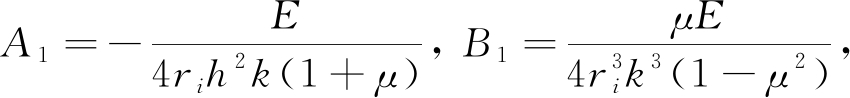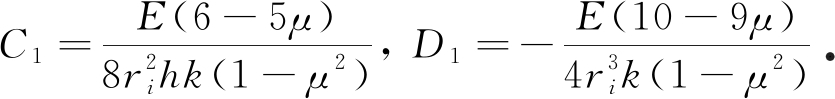2) 环向应力分量σθ的差分方程

(a) r-中心差分，θ-向前差分

σθ(i, j)=(-A2-C2)ψ(i+1, j+2)+(4A2+4C2)ψ(i+1, j+1)+

(-3A2-3C2)ψ(i+1, j)-B2ψ(i, j+3)+(2A2+6B2)ψ(i, j+2)+

(-8A2-12B2+D2)ψ(i, j+1)+(6A2+10B2)ψ(i, j)+

(-3B2-D2)ψ(i, j-1)+(-A2+C2)ψ(i-1, j+2)+

(4A2-4C2)ψ(i-1, j+1)+(-3A2+3C2)ψ(i-1, j)；

(11a)

(b) r-中心差分，θ-向后差分

σθ(i, j)=(3A2+3C2)ψ(i+1, j)+(-4A2-4C2)ψ(i+1, j-1)+

(A2+C2)ψ(i+1, j-2)+(3B2+D2)ψ(i, j+1)+

(-6A2-10B2)ψ(i, j)+(8A2+12B2-D2)ψ(i, j-1)+

(-2A2-6B2)ψ(i, j-2)+B2ψ(i, j-3)+

(3A2-3C2)ψ(i-1, j)+(-4A2+4C2)ψ(i-1, j-1)+

(A2-C2)ψ(i-1, j-2)，

(11b)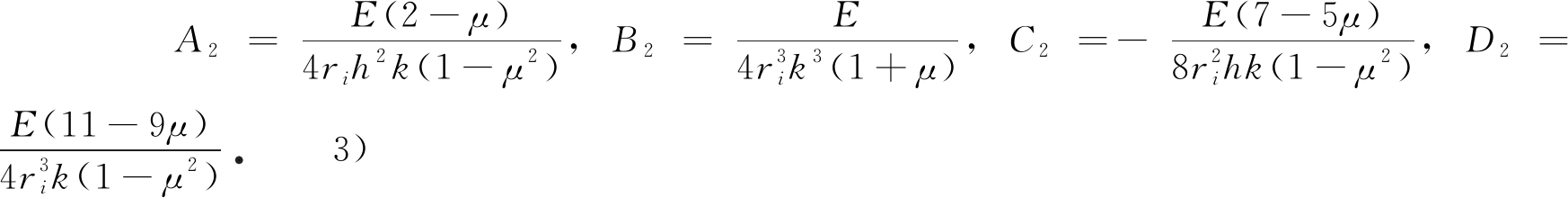剪应力τ的差分方程

(a) r-向前差分，θ-中心差分

τ(i, j)=-A3ψ(i+3, j)-B3ψ(i+2, j+1)+(6A3+2B3)ψ(i+2, j)-

B3ψ(i+2, j-1)+4B3ψ(i+1, j+1)+

(-12A3-8B3+C3+E3)ψ(i+1, j)+4B3ψ(i+1, j-1)+

(-3B3+D3)ψ(i, j+1)+(10A3+6B3-2C3-2D3+F3)ψ(i, j)+

(-3B3+D3)ψ(i, j-1)+(-3A3+C3-E3)ψ(i-1, j)；

(12a)

(b) r-向后差分，θ-中心差分

τ(i, j)=(3A3+C3+E3)ψ(i+1, j)+(3B3+D3)ψ(i, j+1)+

(-10A3-6B3-2C3-2D3+F3)ψ(i, j)+(3B3+D3)ψ(i, j-1)-

4B3ψ(i-1, j+1)+(12A3+8B3+C3-E3)ψ(i-1, j)-

4B3ψ(i-1, j-1)+B3ψ(i-2, j+1)-(6A3+2B3)ψ(i-2, j)+

B3ψ(i-2, j-1)+A3ψ(i-3, j)，

(12b)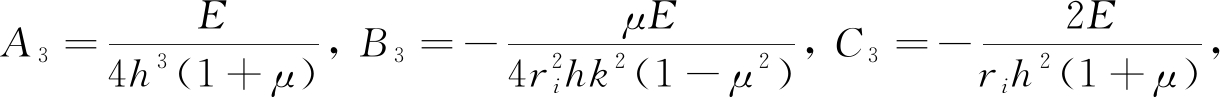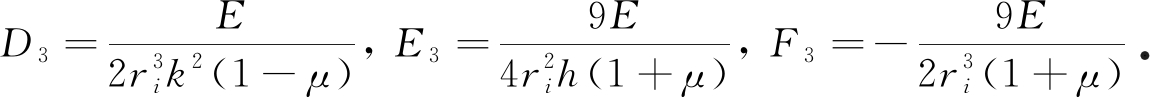## 2 弯曲岩层中的应用

### 2.2 数值计算模型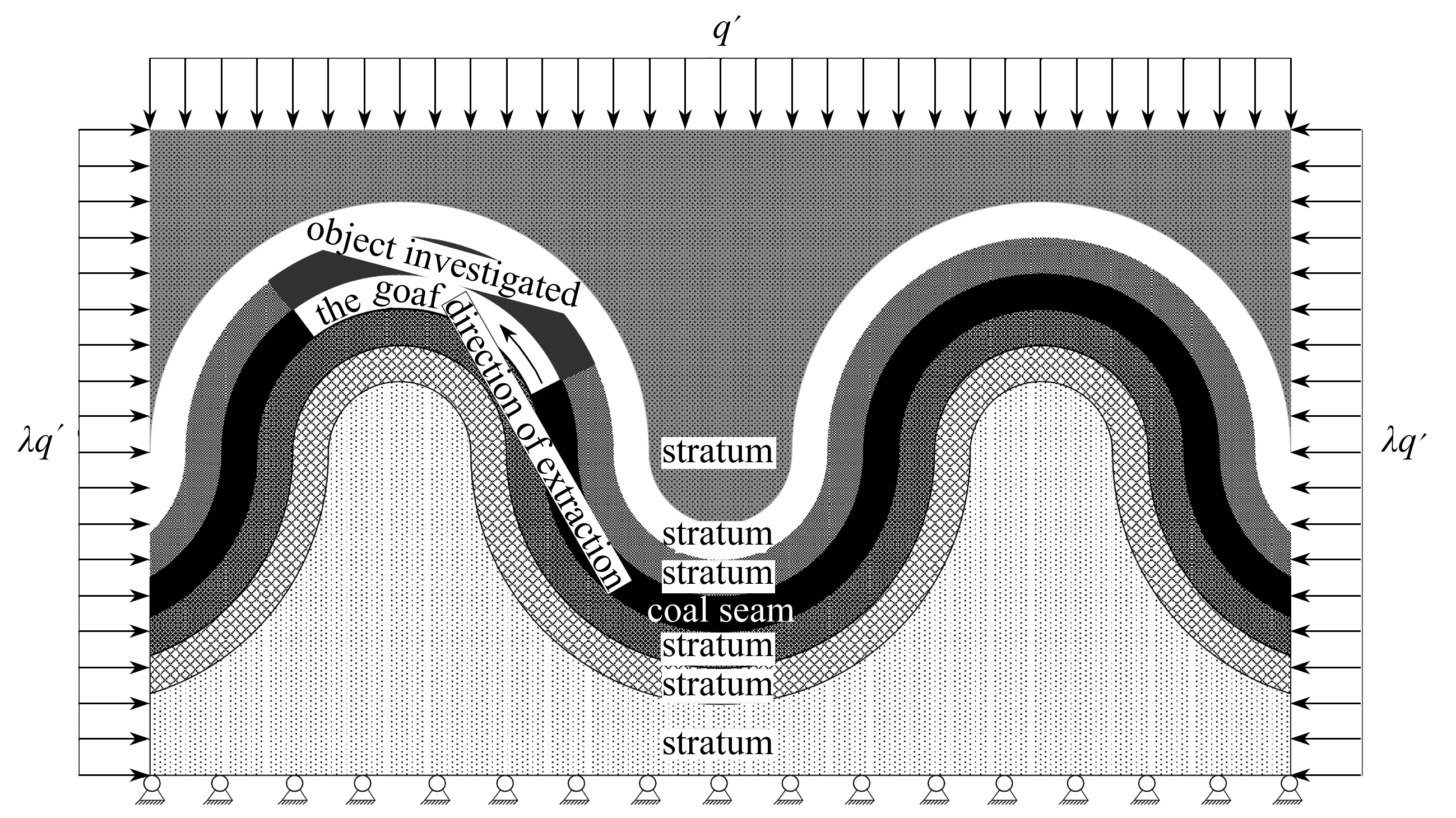Fig. 6 Schematic diagram of coal seam mining in bending rock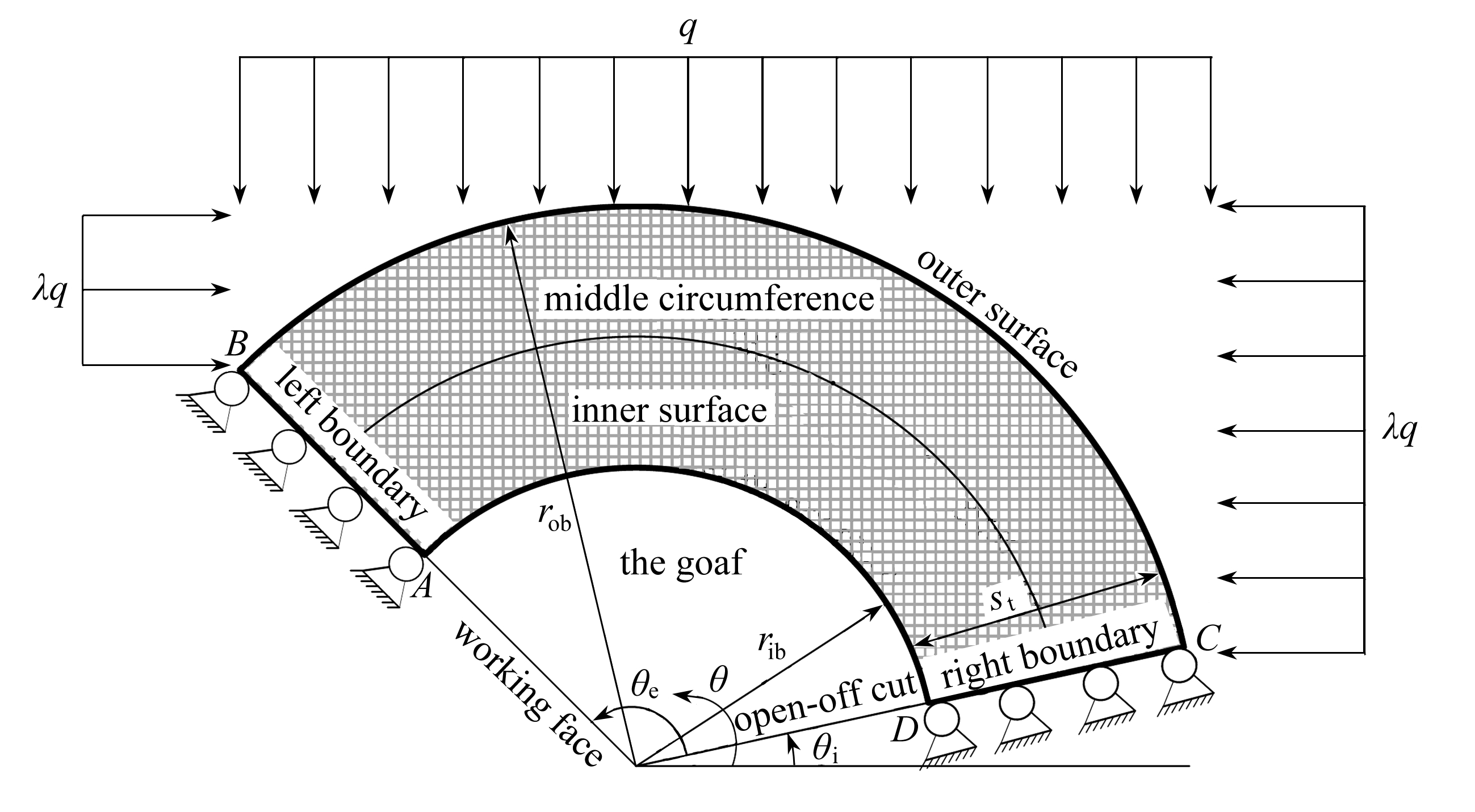Fig. 7 The numerical calculation model for a bending stratum

Table 1 Boundary conditions of the domain for the numerical model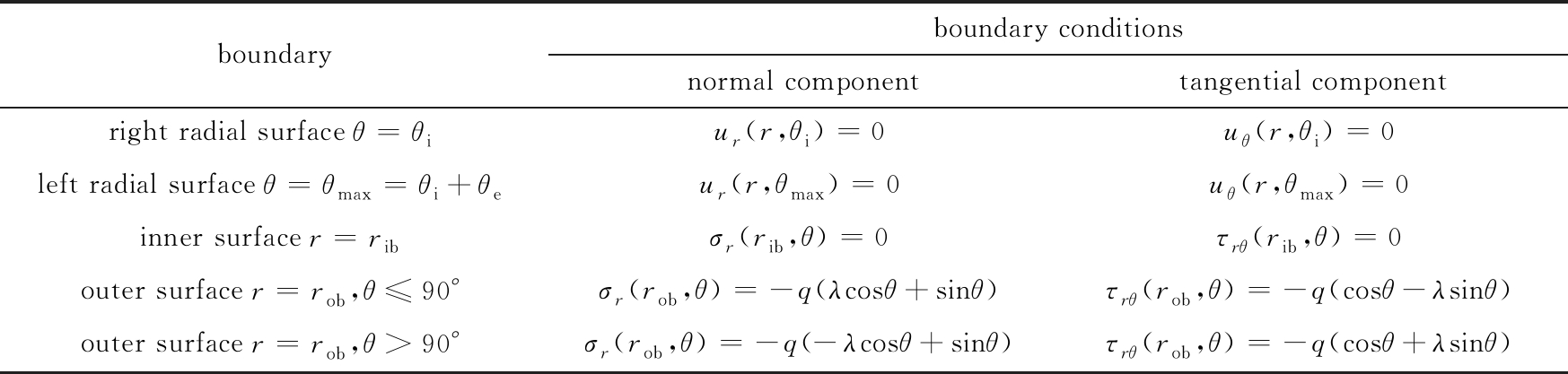boundaryboundary conditionsnormal componenttangential componentright radial surface θ=θiur(r,θi)=0uθ(r,θi)=0left radial surface θ=θmax=θi+θeur(r,θmax)=0uθ(r,θmax)=0inner surfacer=ribσr(rib,θ)=0τrθ(rib,θ)=0outer surface r=rob,θ≤90°σr(rob,θ)=-q(λcosθ+sinθ)τrθ(rob,θ)=-q(cosθ-λsinθ)outer surface r=rob,θ>90°σr(rob,θ)=-q(-λcosθ+sinθ)τrθ(rob,θ)=-q(cosθ+λsinθ)

Table 2 Boundary conditions at the four corner nodes of the domain in the numerical model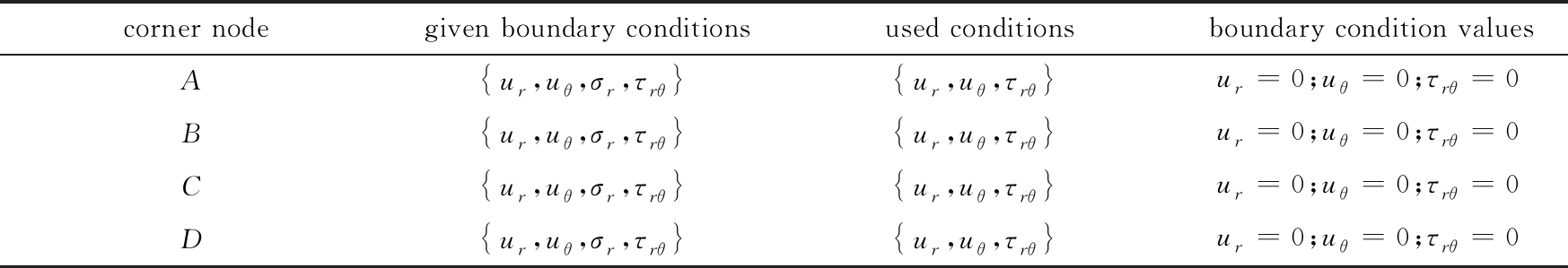corner nodegiven boundary conditionsused conditionsboundary condition valuesA ur,uθ,σr,τrθ ur,uθ,τrθ ur=0;uθ=0;τrθ=0B ur,uθ,σr,τrθ ur,uθ,τrθ ur=0;uθ=0;τrθ=0C ur,uθ,σr,τrθ ur,uθ,τrθ ur=0;uθ=0;τrθ=0D ur,uθ,σr,τrθ ur,uθ,τrθ ur=0;uθ=0;τrθ=0

Table 3 Physical and mechanical properties of rock strata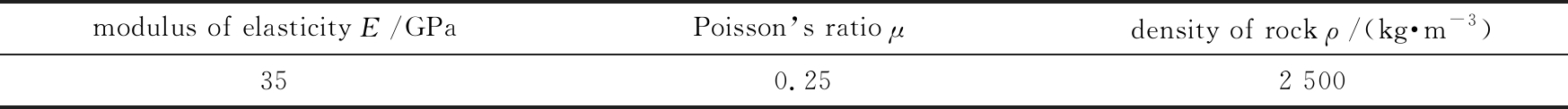modulus of elasticity E/GPaPoisson’s ratio μdensity of rock ρ/(kg·m-3)350.252 500

### 2.3 弯曲覆岩位移分布特征

2.3.1 径向位移分布特征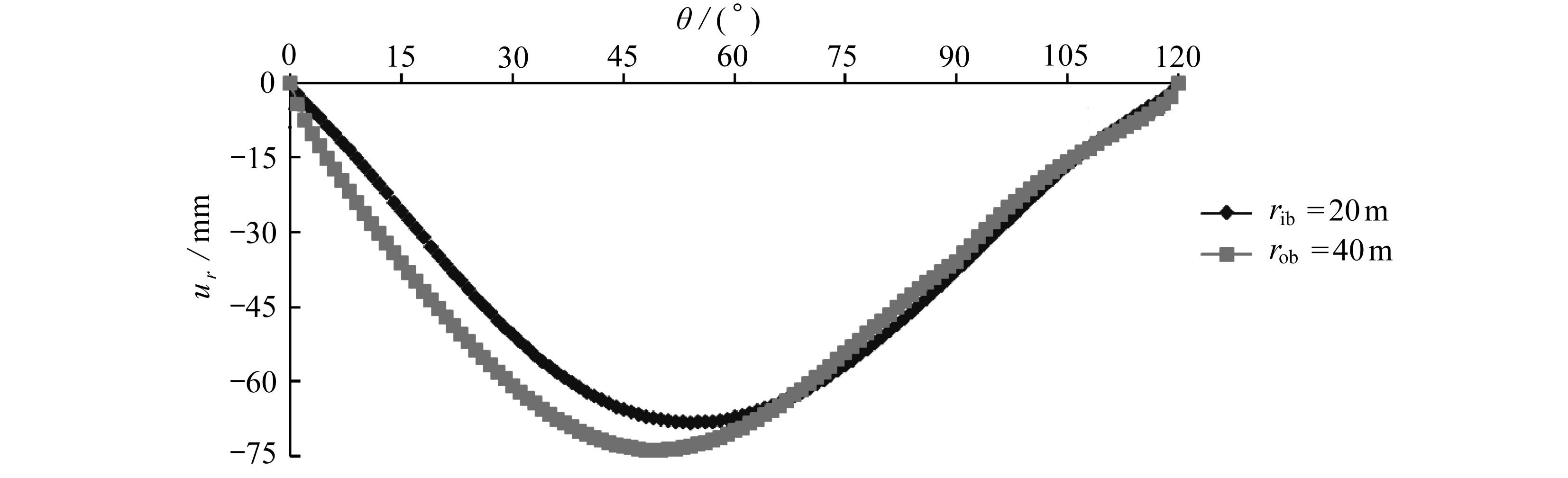Fig. 8 The distribution of radial displacements on inner and outer surfaces along circumferential directions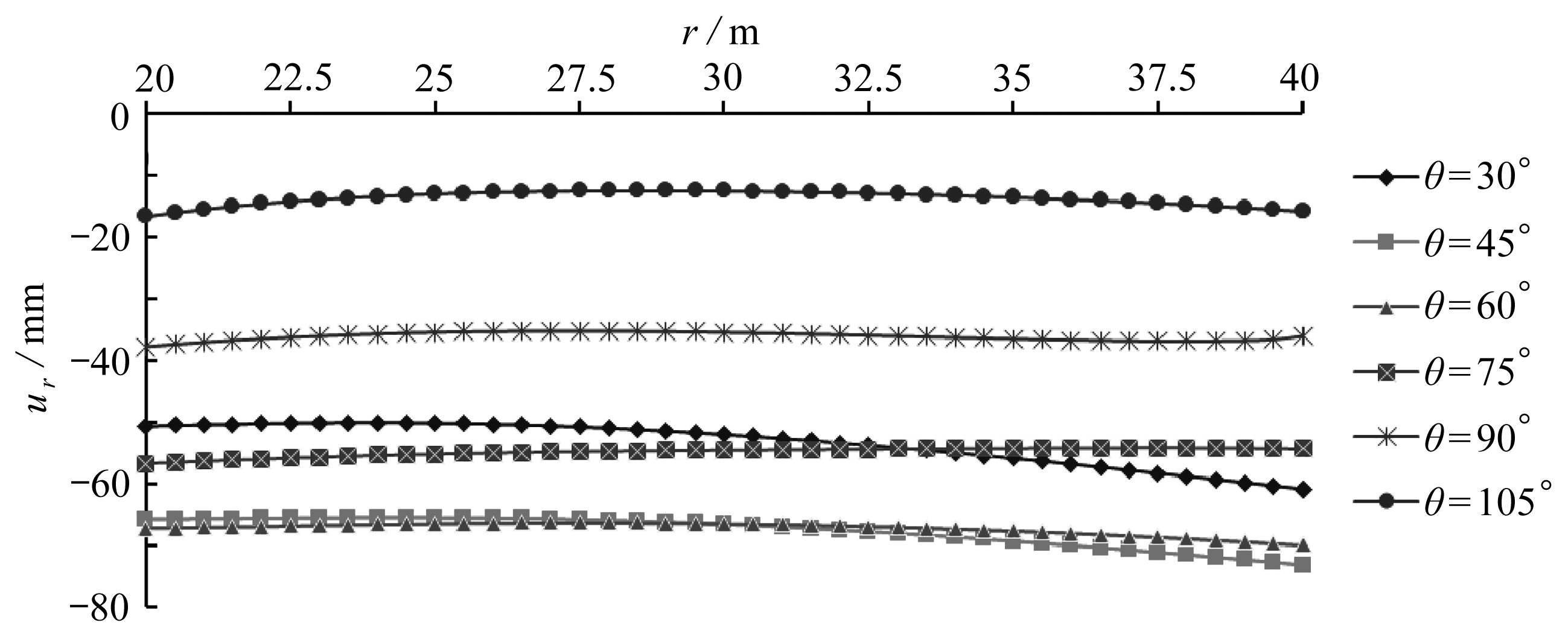Fig. 9 The distribution of radial displacements along radial directions on 6 different sections

2.3.2 环向位移分布特征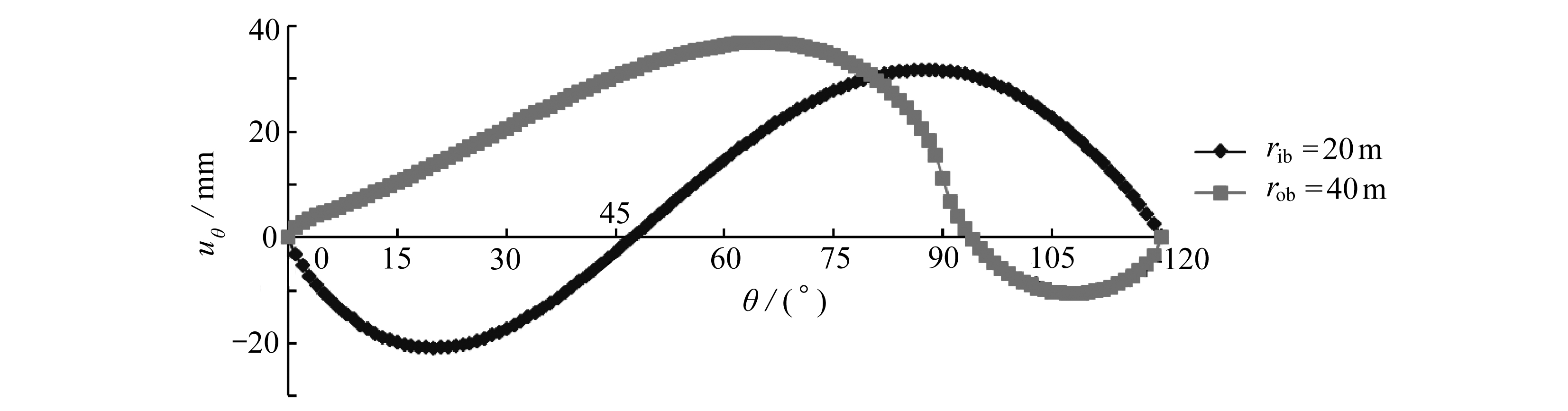Fig. 10 The distribution of circumferential displacements on inner and outer surfaces along circumferential directions

### 2.4 弯曲覆岩应力分布特征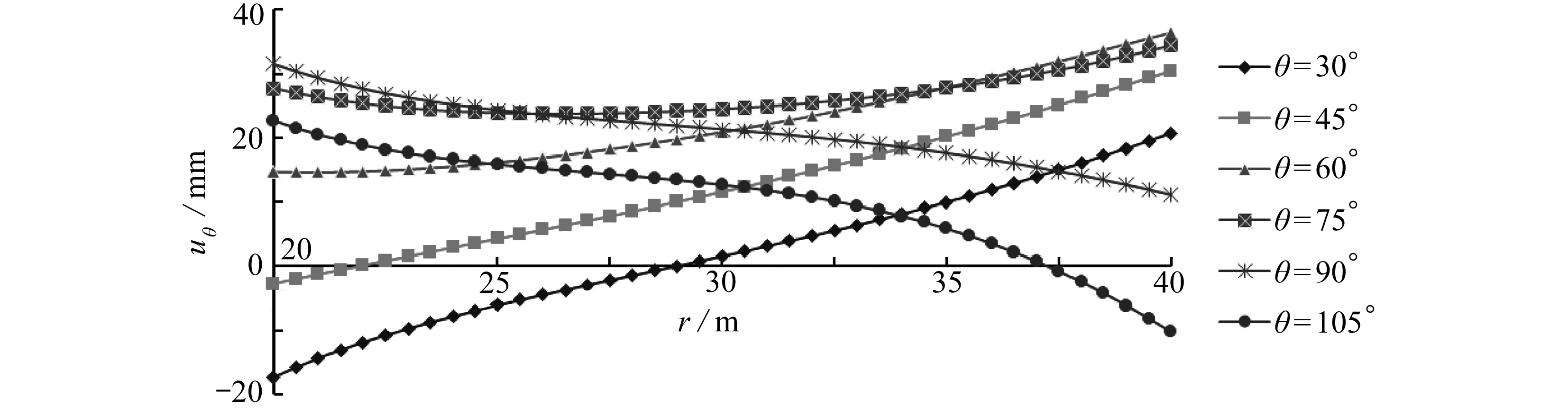Fig. 11 The distribution of circumferential displacements along radial directions on 6 different sections

2.4.1 径向应力分布特征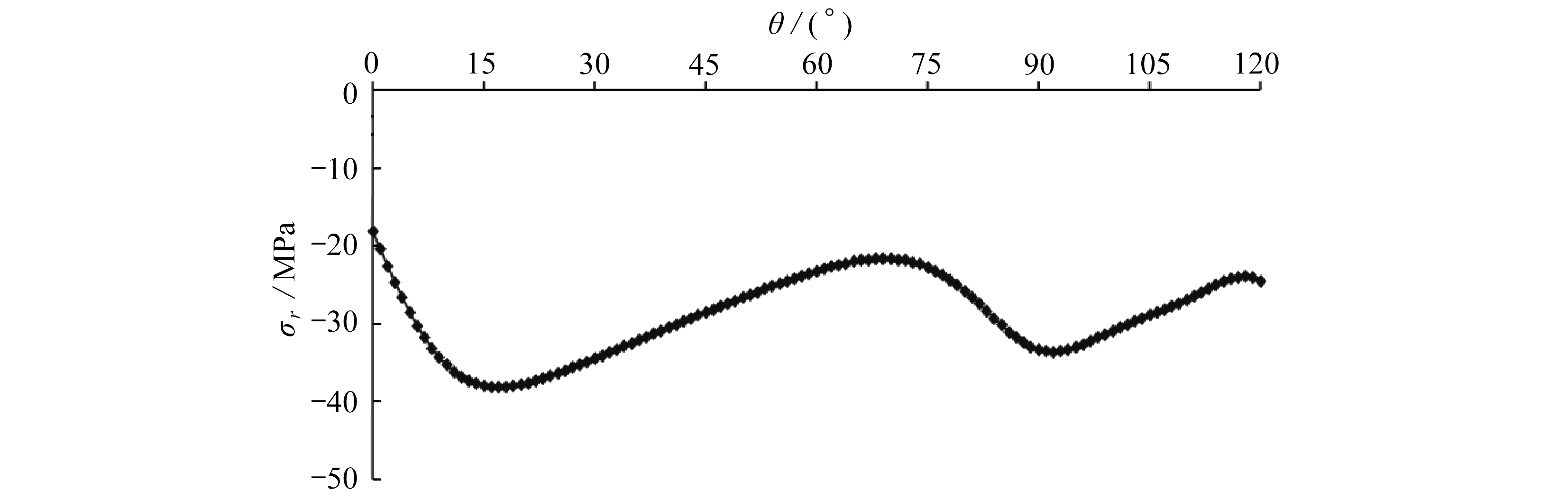Fig. 12 The distribution of radial stresses along the middle circumference

2.4.2 环向应力分布特征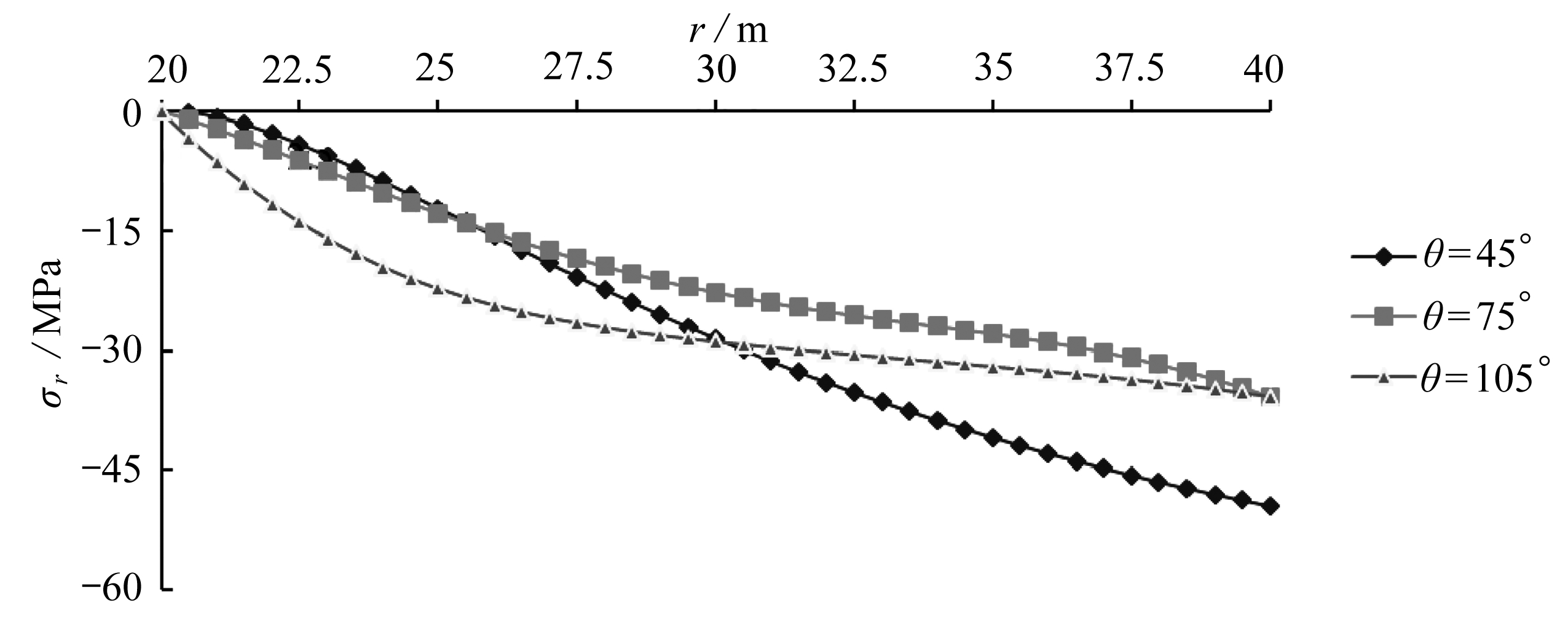Fig. 13 The distribution of radial stresses on 3 different sections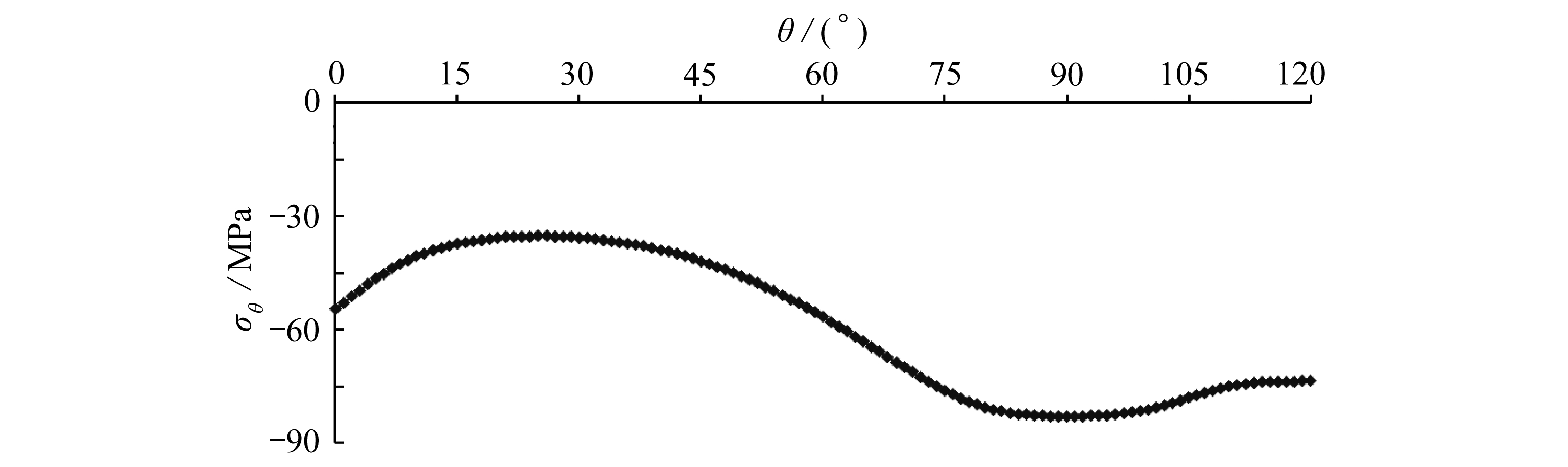Fig. 14 The distribution of circumferential stresses along the middle circumference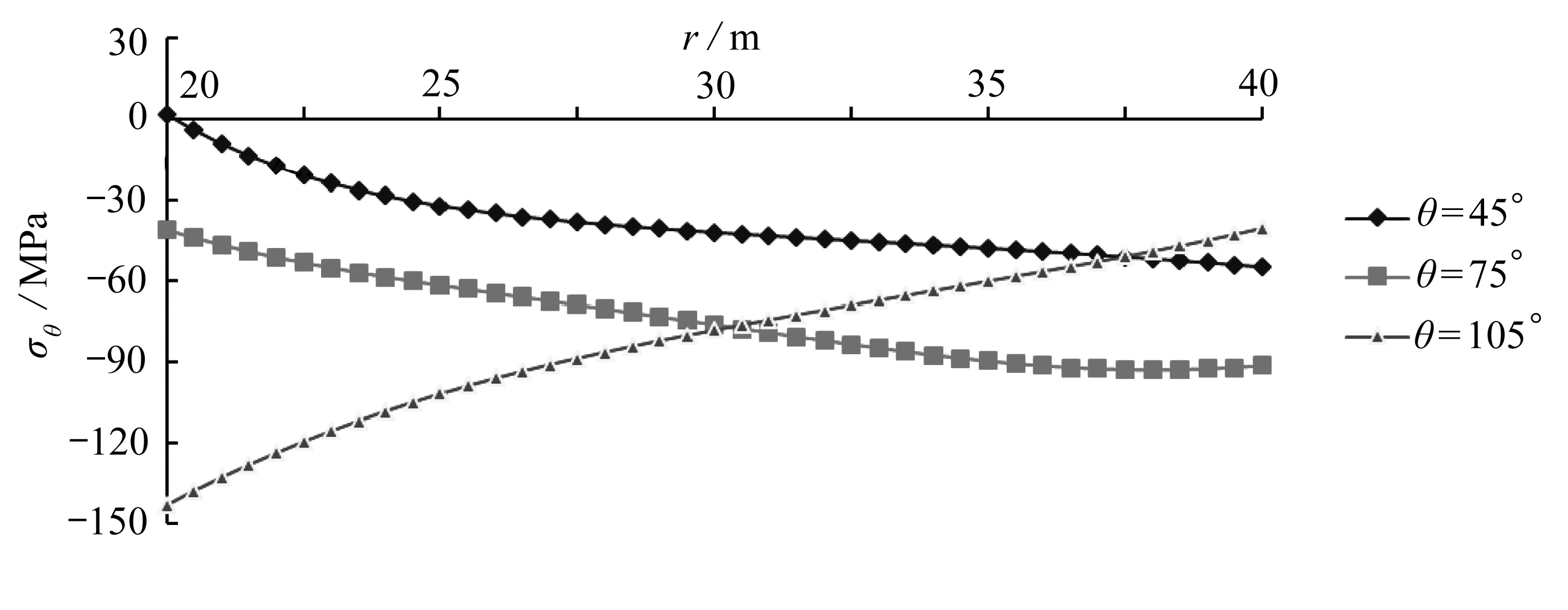Fig. 15 The distribution of circumferential stresses on 3 different sections

2.4.3 剪应力分布特征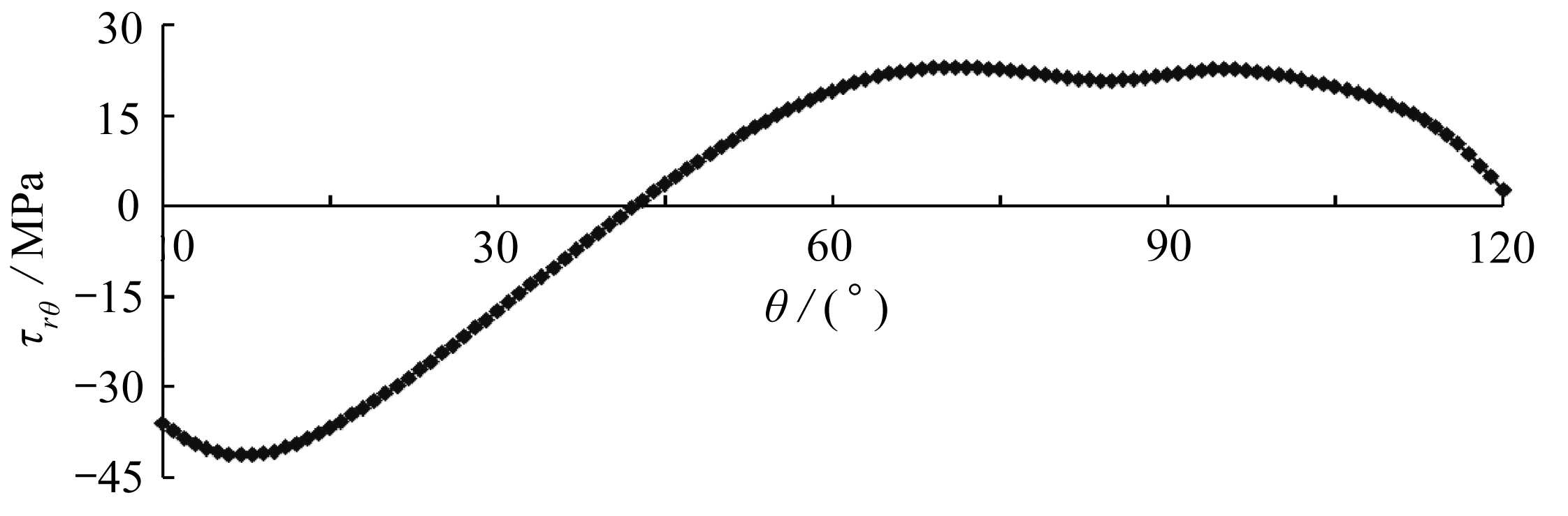Fig. 16 The distribution of shear stresses along the middle circumference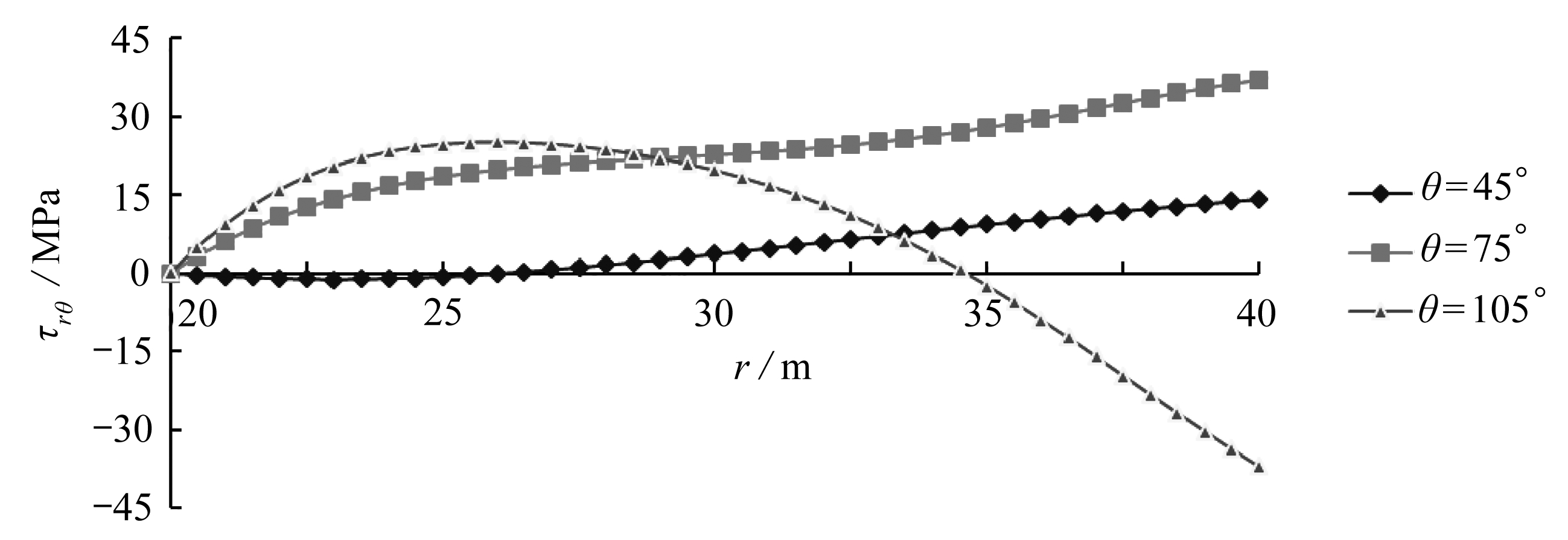Fig. 17 The distribution of shear stresses on 3 different sections

## 3 结 论

1) 通过引入位移函数，得到了极坐标下平面弹性问题位移函数控制方程．通过理论分析、推导，得到了用位移函数表示的位移分量和应力分量．在此基础上，采用差分原理编制程序．

2) 煤层开采后弯曲覆岩的径向位移沿环向呈下凸形状，说明工作面的开采过程中上覆岩层产生下沉变形；弯曲岩层环向位移沿环向既有负值也有正值，且环向位移正值占主要部分．径向应力沿环向分布不均匀，且距离切眼不远处径向应力将达到峰值，径向应力沿径向由内边界向外边界逐渐增大．环向应力沿环向分布也不均匀，较大的环向应力容易引起环向破断．剪应力沿环向的分布也不均匀，距离切眼不远处剪应力将达到峰值，对于小角度的截面上剪应力沿径向由内边界向外边界基本上逐渐增大．

 虎维岳, 何满潮. 深部煤炭资源及开发地质条件研究现状与发展趋势[M]. 北京: 煤炭工业出版社, 2008.(HU Weiyue, HE Manchao. The Present Situation and Development Trend for Deep Coal Resources and the Development of Geological Conditions[M]. Beijing: Coal Industry Publishing House, 2008.(in Chinese))

 何满朝, 钱七虎. 深部岩体力学基础[M]. 北京: 科学出版社, 2010.(HE Manchao, QIAN Qihu. Mechanical Foundation for Deep Rock Mass[M]. Beijing: Science Press, 2010.(in Chinese))

 ODEN J T, RIPPERGER E A. Mechanics of Elastic Structures[M]. 2nd ed. New York: McGraw-Hill Book Company, 1981.

 TIMOSHENKO S P, GOODIER J N. Theory of Elasticity[M]. New York: McGraw-Hill Book Company, 1979.

 CHIANESE R B, ERDLAC R J. The general solution to the distribution of stresses in a circular ring compressed by two forces acting along a diameter[J]. The Quarterly Journal of Mechanics and Applied Mathematics, 1988, 41(2): 239-247.

 BAGCI C. A new unified strength of materials solution for stresses in curved beams and rings[J]. Journal of Mechanical Design, 1992, 114(2): 231-237.

 BAGCI C. Exact elasticity solutions for stresses and deflections in curved beams and rings of exponential and T-sections[J]. Journal of Mechanical Design, 1993, 115(3): 346-358.

 COOK R D. Circumferential stress in curved beams[J]. Journal of Aplied Mechanics, 1992, 59(1): 224-225.

 TUTUNCU N. Plane stress analysis of end-loaded orthotropic curved beams of constant thickness with applications to full rings[J]. Journal of Mechanical Design, 1998, 120(2): 368-374.

 SLOBODA A, HONARMANDI P. Generalized elasticity method for curved beam stress analysis: analytical and numerical comparison for a lifting hook[J]. Mechanics Based Design of Structures and Machines, 2007, 35(3): 319-332.

 钟万勰, 徐新生, 张洪武. 弹性曲梁问题的直接法[J]. 工程力学, 1996, 13(4): 1-8.(ZHONG Wanxie, XU Xinsheng, ZHANG Hongwu. On a direct method for the problem of elastic curved beams[J]. Engineering Mechanics, 1996, 13(4): 1-8.(in Chinese))

 李锐, 田宇, 郑新然, 等. 求解弹性地基上自由矩形中厚板弯曲问题的辛-叠加方法[J]. 应用数学和力学, 2018, 39(8): 875-891.(LI Rui, TIAN Yu, ZHENG Xinran, et al. A symplectic superposition method for bending problems of free-edge rectangular thick plates resting on elastic foundations[J]. Applied Mathematics and Mechanics, 2018, 39(8): 875-891.(in Chinese))

 侯永康, 段树金, 安蕊梅. 满足断裂过程区裂纹张开位移条件应力函数的半解析解法[J]. 应用数学和力学, 2018, 39(8): 979-988.(HOU Yongkang, DUAN Shujin, AN Ruimei. A semi-analytical method for stress functions meeting crack opening displacements in fracture process zones[J]. Applied Mathematics and Mechanics, 2018, 39(8): 979-988.(in Chinese))

 DURELLI A J, RANGANAYAKAMMA B. Parametric solution of stresses in beams[J]. Journal of Engineering Mechanics, 1989, 115(2): 401-415.

 AHMED S R, IDRIS A B M, UDDIN M W. Numerical solution of both ends fixed deep beams[J]. Computers & Structures, 1996, 61(1): 21-29.

 AHMED S R, KHAN M R, ISLAM K M S, et al. Investigation of stresses at the fixed end of deep cantilever beams[J]. Computers & Structures, 1998, 69(3): 329-338.

 姚晓东, 张元海. 基于抽象翘曲位移函数的箱形梁剪力滞效应分析[J]. 应用数学和力学, 2018, 39(12): 1351-1363.(YAO Xiaodong, ZHANG Yuanhai. Analysis of shear lag effects in box girders based on abstract warping displacement functions[J]. Applied Mathematics and Mechanics, 2018, 39(12): 1351-1363.(in Chinese))

 COOK R D. Axisymmetric finite element analysis for pure moment loading of curved beams and pipe bends[J]. Computers & Structures, 1989, 33(2): 483-487.

 RATTANAWANGCHAROEN N, BAI H, SHAH A H. A 3D cylindrical finite element model for thick curved beam stress analysis[J]. International Journal for Numerical Methods in Engineering, 2004, 59(4): 511-531.

 RICHARDS T H, DANIELS M J. Enhancing finite element surface stress predictions: a semi-analytic technique for axisymmetric solids[J]. The Journal of Strain Analysis for Engineering Design, 1987, 22(2): 75-86.

 SMART J. On the determination of boundary stresses in finite elements[J]. The Journal of Strain Analysis for Engineering Design, 1987, 22(2): 87-96.

 GANGAN P. The curved beam/deep arch/finite ring element revisited[J]. International Journal for Numerical Methods in Engineering, 1985, 21(3): 389-407.

 DOW J O, JONES M S, HARWOOD S A. A new approach to boundary modeling for finite difference applications in solid mechanics[J]. International Journal for Numerical Methods in Engineering, 1990, 30(1): 99-113.

 RANZI G, GARA F, LEONI G, et al. Analysis of composite beams with partial shear interaction using available modelling techniques: a comparative study[J]. Computers & Structures, 2006, 84(13/14): 930-941.

 AHMED S R, NATH S K D, UDDIN M W. Optimum shapes of tire-treads for avoiding lateral slippage between tires and roads[J]. International Journal for Numerical Methods in Engineering, 2005, 64(6): 729-750.

 AHMED S R, HOSSAIN M Z, UDDIN M W. A general mathematical formulation for finite-difference solution of mixed-boundary-value problems of anisotropic materials[J]. Computers & Structures, 2005, 83(1): 35-51.

 HOSSAIN M Z, AHMED S R, UDDIN M W. Generalized mathematical model for the solution of mixed-boundary-value elastic problems[J]. Applied Mathematics and Computation, 2005, 169(2): 1247-1275.

 XU W, LI G. Finite difference three-dimensional solution of stresses in adhesively bonded composite tubular joint subjected to torsion[J]. International Journal of Adhesion and Adhesives, 2010, 30(4): 191-199.

 NATH S K D, AHMED S R. A displacement potential-based numerical solution for orthotropic composite panels under end moment and shear loading[J]. Journal of Mechanics of Materials and Structures, 2009, 4(6): 987-1004.

 MCCORQUODALE P, COLELLA P, GROTE D P, et al. A node-centered local refinement algorithm for Poisson’s equation in complex geometries[J]. Journal of Computational Physics, 2004, 201(1): 34-60.

 DING H, SHU C. A stencil adaptive algorithm for finite difference solution of incompressible viscous flows[J]. Journal of Computational Physics, 2006, 214(1): 397-420.

 MIN C, GIBOU F, CENICEROS H D. A supra-convergent finite difference scheme for the variable coefficient Poisson equation on non-graded grids[J]. Journal of Computational Physics, 2006, 218(1): 123-140.

 BIENIASZ L K. Experiments with a local adaptive grid h-refinement for the finite-difference solution of BVPs in singularly perturbed second-order ODEs[J]. Applied Mathematics and Computation, 2008, 195(1): 196-219.

# Analysis on Deformation and Stress of Bending Stratum Based on the Elastic Theory for Curved Beams

BU Wankui1, XU Hui1, ZHAO Yucheng2

(1. College of Urban Construction, Heze University, Heze, Shandong 274015, P.R.China; 2. School of Mechanics and Civil Engineering, China University of Mining and Technology, Xuzhou, Jiangsu 221116, P.R.China)

Abstract: A displacement function suitable for plane curved beams in polar coordinates was introduced, and the partial differential governing equation for plane curved beams was obtained through theoretical analysis. Then, the displacement components and stress components were formulated with the displacement function. On this basis, the finite difference schemes of the partial differential governing equation, the displacement components and the stress components for the curved beam in polar coordinates were presented. Finally, these theoretical formulas were applied to analyze the displacement and stress distributions of the bending stratum. The results indicate that: 1) The bending stratum sinks down after excavation of the coal seam, and there are both tension and compression in the circumferential direction. 2) The radial stress reaches a peak value not far from the open-off cut and increases gradually from the inner surface to the outer surface along the radial direction; the circumferential stress reaches the peak value not far behind the working face and may cause circumferential compressive fracture in the bending stratum; the shear stress reaches the peak value not far from the open-off cut and increases from the inner surface to the outer surface along the radial direction for small-angle sections. The work provides a scientific basis and reference for coal mining engineering.

Key words: curved beam; theory of elasticity; finite difference; deformation of overburden rock; stress analysis

ⓒ 应用数学和力学编委会，ISSN 1000-0887

http://www.applmathmech.cn

*收稿日期： 2019-03-01； 修订日期：2019-04-02

DOI: 10.21656/1000-0887.400081

Foundation item: The National Natural Science Foundation of China(General Program)(51574228)